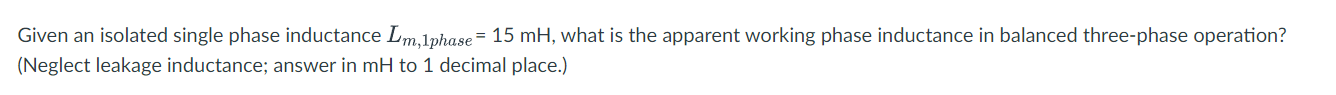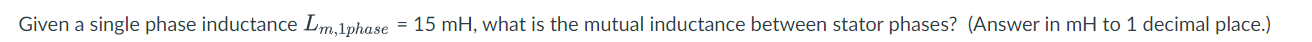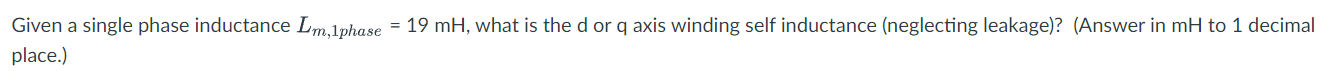Home / Expert Answers / Electrical Engineering / given-an-isolated-single-phase-inductance-lm-1phase-15mh-what-is-the-pa711

# (Solved): Given an isolated single phase inductance Lm,1phase=15mH, what is the ...​​​​​​​

Given an isolated single phase inductance , what is the apparent working phase inductance in balanced three-phase operation? (Neglect leakage inductance; answer in to 1 decimal place.) Given a single phase inductance , what is the or q axis winding self inductance (neglecting leakage)? (Answer in to 1 decimal place.)

We have an Answer from Expert

1. In a balanced three-phase operation, the apparent working phase inductance can be determined using the formula:

Lapparent =

Substituting the given value of Lim = 15 mH into the formula:

Lapparent = 15 mH / ?3 ? 8.7 mH

Therefore, the apparent working phase inductance in balanced three-phase operation is approximately 8.7 mH.

We have an Answer from Expert